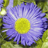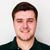# Percentage Change Calculation

1150
4
06-12-2020 01:12 PMOccasional Contributor

Trying to calculate percentage change in numbers from year 2019 to 2020. The calculation in the field calculator should be 2020 - 2019 = divided by 2019. I have tried several ways but it is not showing the correct answer. Here is what I have 4244 - 6363 = -2119 / 6363 shows -.33 percent change. [Volume_2020] - [Volume_2019] / [Volume_2019] but it gives me the answer of 4243. Am I missing same quotations?

Tags (2)
1 Solution

Accepted SolutionsbyEsri Contributor

Hi Michelle,

The first portion of the formula needs to be in parentheses like this:

([Volume_2020] - [Volume_2019]) / [Volume_2019]

Otherwise, the division will be performed before the subtraction due to order of operations. Let me know if this works!

Best,

Josh

4 RepliesbyEsri Contributor

Hi Michelle,

The first portion of the formula needs to be in parentheses like this:

([Volume_2020] - [Volume_2019]) / [Volume_2019]

Otherwise, the division will be performed before the subtraction due to order of operations. Let me know if this works!

Best,

JoshOccasional Contributor

Thanks. I was using brackets not parentheses. I’ll try that.Occasional Contributor

It didn’t work. It said it didn’t like the Type Mismatch.Occasional Contributor

It worked. For some reason, it was not working inside my geodatabase. So I took it out and then did the calculation. Thanks for the help.